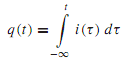## Explain current and magnetic force, Electrical Engineering

Assignment Help:

Q. Explain Current and Magnetic Force?

The rate ofmovement of net positive charge per unit of time through a cross section of a conductor is known as current,

i(t) = dq / dt

The SI unit of current is the ampere (A), which represents 1 coulomb per second. In most metallic conductors, such as copper wires, current is exclusively the movement of free electrons in the wire. Since electrons are negative, and since the direction designated for the current is that of the net positive charge movement, the charges in the wire are thus moving in the direction opposite to the direction of the current designation. The net charge transferred at a particular time is the net area under the current-time curve from the beginning of time to the present,While Coulomb's law has to do with the electric force associated with two charged bodies, Ampere's lawof force is concerned with magnetic forces associatedwith two loops ofwire carrying currents by virtue of the motion of charges in the loops. Note that isolated current elements do not exist without sources and sinks of charges at their ends; magnetic monopoles do not exist.

#### Explain biased and double clipper circuits, Q. Explain biased and double c...

Q. Explain biased and double clipper circuits.   Clipper can be either forward biased or reverse biased. If a battery is connected in series with the diode in a positi

#### Sketch a graph comparing the fcc class, a) In the United States, the Federa...

a) In the United States, the Federal Communications Commission (FCC) is charged with the regulation of radio and wire communication (FCC part 15). It basically sets limits on the

#### Sketch the resulting transfer curves, Q. Consider the MOSFET circuit with v...

Q. Consider the MOSFET circuit with variable voltage, with RD = 2k and VDD = 12 V. The static characteristics of the n-channel enhancement MOSFET are given in Figure. (a) Drawt

#### amp circuit , Refer to the op amp circuit shown below. Let R 1 = ...

Refer to the op amp circuit shown below. Let R 1 = 140 k_, R 2 = 10 k_, R 3 = 10 k_, R 4 = 20 k_, RL = 10 k_. Assume the op amp is ideal. Find the equation relating the

#### Amplifiers with feedback, Q. Amplifiers with Feedback? Almost all pract...

Q. Amplifiers with Feedback? Almost all practical amplifier circuits include some form of negative feedback. The advantages gained with feedback may include the following: •

#### Emi, what is strain gage

what is strain gage

Q. Explain Steady-State Error of Linear Systems? If the steady-state response of the output does not agree exactly with the steady state of the input, the system is said to hav

#### Jump instruction - branch operations , Jump Instruction There are two ...

Jump Instruction There are two types of jump  instruction unconditional jump conditional jumps

#### Define some applications of digital counters, Define some Applications of D...

Define some Applications of Digital Counters? Digital counters are extremely useful in many applications. They can be effortlessly found in digital clocks and parallel-to-seri

#### General, a single phase 120 volts, 60hertz supply is connected to a coil of...

a single phase 120 volts, 60hertz supply is connected to a coil of 200 turns wound round a toroidal magnetic core with a mean length 100cm and cross section 20cm square &relative p